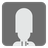All the Same
Simple
JA RU English UK ZH-HANS
We have prepared a set of Editor's Choice Solutions. You will see them first after you solve the mission. In order to see all other solutions you should change the filter.

In this mission you should check if all elements in the given list are equal.

Input: List.

Output: Bool.

Example:

```all_the_same([1, 1, 1]) == True
all_the_same([1, 2, 1]) == False
all_the_same(['a', 'a', 'a']) == True
all_the_same([]) == True
```

The idea for this mission was found on Python Tricks series by Dan Bader

Precondition: all elements of the input list are hashable